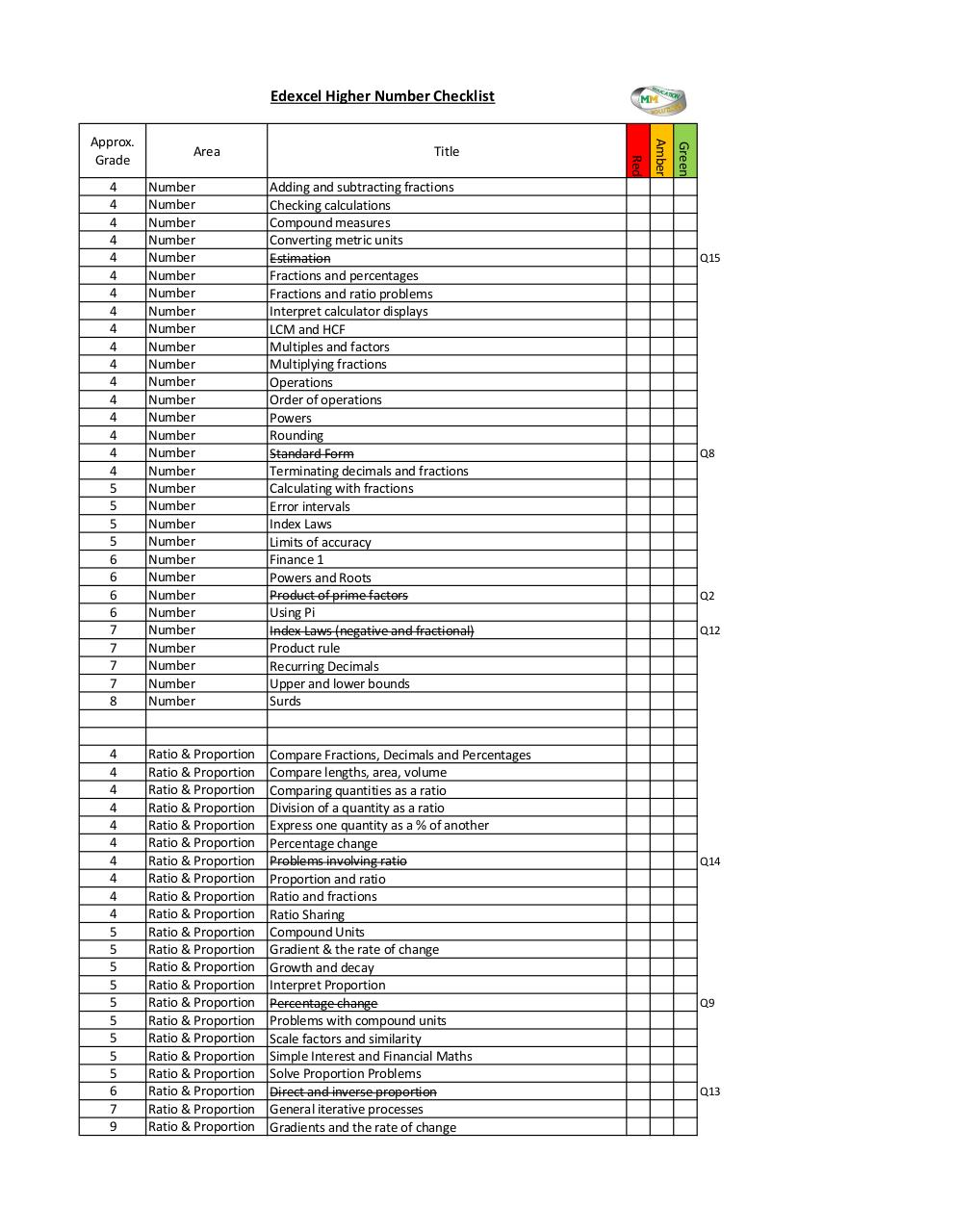# Number (Post Paper 1) .pdf

### File information

Original filename: Number (Post Paper 1).pdf
Title: Checklist (Post Paper 1).xlsx
Author: Michael

This PDF 1.7 document has been generated by / Microsoft: Print To PDF, and has been sent on pdf-archive.com on 25/05/2017 at 16:01, from IP address 188.222.x.x. The current document download page has been viewed 172 times.
File size: 342 KB (1 page).
Privacy: public file

Number (Post Paper 1).pdf (PDF, 342 KB)

### Document preview

Edexcel Higher Number Checklist

4
4
4
4
4
4
4
4
4
4
4
4
4
4
4
4
4
5
5
5
5
6
6
6
6
7
7
7
7
8

Number
Number
Number
Number
Number
Number
Number
Number
Number
Number
Number
Number
Number
Number
Number
Number
Number
Number
Number
Number
Number
Number
Number
Number
Number
Number
Number
Number
Number
Number

Checking calculations
Compound measures
Converting metric units
Estimation
Fractions and percentages
Fractions and ratio problems
Interpret calculator displays
LCM and HCF
Multiples and factors
Multiplying fractions
Operations
Order of operations
Powers
Rounding
Standard Form
Terminating decimals and fractions
Calculating with fractions
Error intervals
Index Laws
Limits of accuracy
Finance 1
Powers and Roots
Product of prime factors
Using Pi
Index Laws (negative and fractional)
Product rule
Recurring Decimals
Upper and lower bounds
Surds

4
4
4
4
4
4
4
4
4
4
5
5
5
5
5
5
5
5
5
6
7
9

Ratio &amp; Proportion
Ratio &amp; Proportion
Ratio &amp; Proportion
Ratio &amp; Proportion
Ratio &amp; Proportion
Ratio &amp; Proportion
Ratio &amp; Proportion
Ratio &amp; Proportion
Ratio &amp; Proportion
Ratio &amp; Proportion
Ratio &amp; Proportion
Ratio &amp; Proportion
Ratio &amp; Proportion
Ratio &amp; Proportion
Ratio &amp; Proportion
Ratio &amp; Proportion
Ratio &amp; Proportion
Ratio &amp; Proportion
Ratio &amp; Proportion
Ratio &amp; Proportion
Ratio &amp; Proportion
Ratio &amp; Proportion

Compare Fractions, Decimals and Percentages
Compare lengths, area, volume
Comparing quantities as a ratio
Division of a quantity as a ratio
Express one quantity as a % of another
Percentage change
Problems involving ratio
Proportion and ratio
Ratio and fractions
Ratio Sharing
Compound Units
Gradient &amp; the rate of change
Growth and decay
Interpret Proportion
Percentage change
Problems with compound units
Scale factors and similarity
Simple Interest and Financial Maths
Solve Proportion Problems
Direct and inverse proportion
General iterative processes
Gradients and the rate of change

Green

Title

Amber

Area

Red

Approx.

Q15

Q8

Q2
Q12

Q14

Q9

Q13#### HTML Code

Copy the following HTML code to share your document on a Website or Blog

#### QR Code### Related keywords